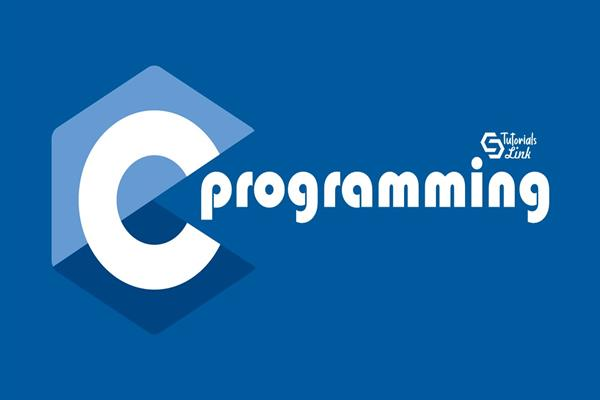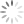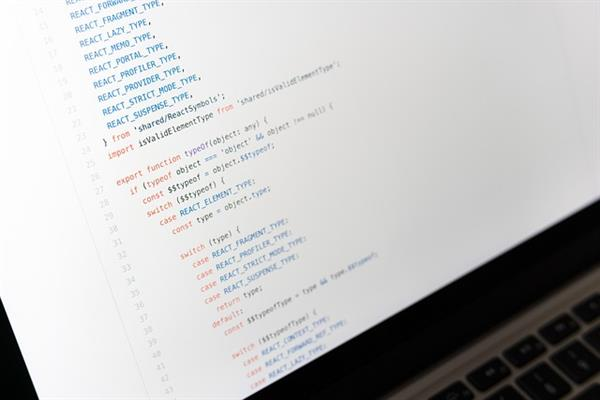Loading, please wait...# Shortest Common Super-Sequence In Dynamic Programming

In this blog, we'll discuss about the shortest common super-sequence in dynamic programming.

The super-sequences are part of the computer science problem. Here you will be given two strings, say S1 & S2. So, through this program, you have to create a string that includes both the strings, i.e. S1 and S2. Therefore, both the string's value combines to make a super-sequence.

Let's take an example, suppose there are two strings ‘Coding’ and ‘Ninja’. Then the shortest common super-sequence among these two strings will be ‘in’.

Repeating this dynamic programming, you can print shortest common supersequence. Now, let's learn more about the super-sequence in dynamic programming.

## What is super-sequence in dynamic programming?

Mainly used in the C or dynamic programming of computer science, the super-sequence creates a sequence where both elements are present. So, the two strings provided to you are the sub-sequence of the Shortest Common Super-Sequence.

Also, for the sequences A and B, the shortest sequence will be the subsequence of A and B. Therefore, to print shortest common supersequence is the most extended problem in the common sequence topic.

For example: Let's take two strings and name them A1 and B1. Now, let's provide some values to both strings.

A1 = Red and B1 = Bed

Both values of strings A1 and B1 will be combined to make a super-sequence.

RedBed ( common super-sequence)

Here we see the process of extracting a common super-sequence from the two given strings. First, however, we have to find the shortest common super-sequence.

To find the shortest common super-sequence, we'll make the string that contains both values of A1 and B1.

We are calling this string "RBED" (shortest common super-sequence).

We achieved a string that consists of both values of a common super-sequence and simultaneously saved the letter count. So, our initial string, "RedBed", has six letters. On the other hand, our new shortest super-sequence, "RBED", has only four letters.

We have only understood deriving the shortest super-sequence in dynamic programming. So let's understand the method in detail as it will help you implement zigzag traversal of binary trees.

## How to find the shortest super-sequence?

To understand the process of finding the shortest super-sequence, we'll take the help of two different strings.

Let's take S1 and S2 (names of the two strings) where S1 = White and S2 = Right.

Now, let's create the shortest super-sequence of these strings, i.e. Whrighte. In the "Whrighte", both strings' value is present. Therefore, we can extract the length of the shortest super-sequence. Here's how you can do that.

Let's start making the shortest super-sequence for the words White and Right. Here, we'll create a string with W, R, and E as the uncommon values and I, T, and H as the expected values. So, why don't we write a string that eliminates the common values? Indeed, we can do that. Therefore, we made a string that consisted of the standard letters of both string's values and got "WHRIGTE."

The Longest Common Sequence calculates the standard letters in the string values S1 and S1. With the help of LCS, we used I, T, and H once from both sequences. Thus, we can calculate the string length by:

S1 + S1 - Length of LCS.

As a result, we get,

The length of S1 is five, and the Length of S2 is 5. The length of the longest common sequence (LCM) is 3.

So, 5 + 5 = 10. Subtracting the number by LCM, we get,

10 - 3 = 7. It means that the length of our shortest super-sequence is 7.

Now, we understand how it is possible to find the length of the shortest common super-sequence. Let's get you through the process of printing the shortest common super-sequence.

## How to print the shortest common super-sequence?

We have now figured out the basic concept of finding the shortest common super-sequence length. You can see that quickly, but first, you must understand the Longest Common Sequence because before printing the shortest super-sequence, you must know its length.

### Dynamic programming

In dynamic programming, the compiler stores all the string values, also known as memorization.

Therefore, many programmers use the DP technique to understand how the compiler behaves when we execute a program.

It is memorized in i and j, where i runs horizontally and j runs vertically. Both values have 0 in the beginning. When your compiler gets the common sequence between both the strings, it counts them as one and keeps adding the number if found more common letters.

As we now know the length of our shortest super-sequence, we'll understand the quick process to print it.

S1 = White, S2 = Right

while (i>0??j>0)

{

if (s1[i-1] = = s2[j-2])

{

ans+ = s1[i-1];

i--, j--;

}

else if (dp[i-1][j]>dp[i][j-1])

}

else

{

ans+ s2[j-1];

j--

}

}

This is the fundamental problem to print the shortest common super-sequence. Now, let's understand why we used certain code lines to find the shortest super-sequence.

The i>0 tells the compiler that string 1 has some length (S1).

Similarly, the j>0 tells the compiler that string 2 has some length (S2).

Then, we used the most important code, i.e. ans+ = s1[i-1], where the compiler executes the common sequence by comparing both strings.

Same for the string 2, i.e. ans+ s2[j-1].

At the end of the program, we get the result sequence-wise. Then, we use another line of code to reverse the program. It will execute the shortest common super-sequence systematically.

As a result, we get "WHRIGTE."

So, this is how simple it is to execute the shortest common super-sequence program. Now, let's understand where you can use the shortest common super-sequence program.

## Where to execute the shortest super-sequence program?

Deriving the shortest super-sequence program is possible in all dynamic programming, such as C and C++. However, it would help if you remembered specific rule changes while executing the programs. The super-sequence program is also very vital in zigzag traversal of binary tree which is basically the pushing of nodes in a so-called zigzag pattern along the binary tree.

## Conclusion:

The purpose of the shortest common super-sequence program is to find the common letters from both strings and avoid using them twice.

In addition, it reduces the length of the word because now, we use the subsequence of the strings. So, your string's size might be 20, which can be reduced to 15 or 10.

Understand the concept of the shortest common super-sequence and then execute its code in the compiler.